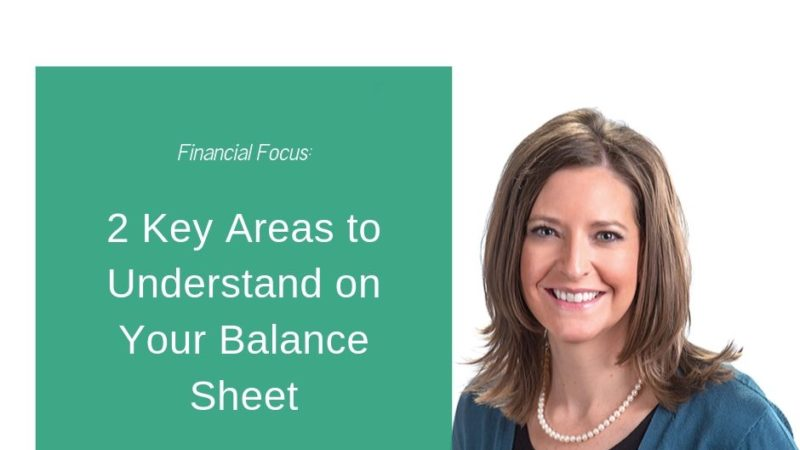Article by Jocelyn Knutson, Senior Loan Officer, Sterling Branch

Let’s take a few minutes to discuss the importance of a balance sheet. An accurate balance sheet is an important tool for producers to use when measuring financial strength and trends, as well as identifying possible areas of weakness. A balance sheet is simply a list of all assets and liabilities AT A POINT IN TIME (date specific). Within the balance sheet, Liquidity and Solvency are two key areas of focus.

Liquidity is the ability to generate cash in the amount needed and at the time it is needed.  Two ways of measuring liquidity are Working Capital and Current Ratio.

• Working Capital is calculated by taking current assets less current liabilities (a \$ value).
• Working Capital should be positive and increase over time.
• It is affected by profit or loss, capital purchase and sales, refinancing debt, changes in operation, etc.
• For example: \$100,000 current assets – \$80,000 current liabilities = \$20,000 working capital
• Current Ratio is calculated by taking current assets divided by current liabilities (a number value).
• An example would be current ratio of 1.5 to 1 or 1.5:1. This means for every \$1.00 of current liabilities, the producer has \$1.50 in current assets to pay it.

Solvency is the ability to pay off all liabilities if all assets were sold.  Ways of measuring solvency include evaluating Net Worth, Owner Equity, Debt to Asset and Debt to Equity.  These measurements or ratios help identify how “leveraged” a borrower is.  The more “leveraged” a borrower is, the harder it may be to overcome adversity (the ability to borrow decreases as leverage increases).

• Net Worth is calculated by taking total assets less total liabilities (a \$ value).
• Owner Equity is calculated by taking net worth divided by total assets (a % value).
• This tells what part of total assets is financed by the owner’s equity capital.
• As an example, if owner equity is 65%, for every \$1 in assets, the borrower has \$0.65 of equity (and \$0.35 of debt).
• Debt to Asset is calculated by taking total liabilities divided by total assets (a % value).
• This tells what part of total assets are financed by debt.
• As an example, if debt to asset ratio is 35%, for every \$0.35 in debt, the borrower has \$1.00 in assets.
• This measurement is OPPOSITE OF OWNER EQUITY.
• Debt to Equity is calculated by taking total liabilities divided by net worth (a % value).
• This tells what proportion of financing is provided by the lender vs. the borrower.
• Smaller numbers are preferred. A number of 0.10 or 10% indicates the borrower has little debt.
• Large values here (less than 1.0 or 100%) represent minimal equity. A value of 0.99 or 99% would mean the borrower has little equity and an increased chance of insolvency.
• A value of 1.0 or 100% indicates lender and borrower are providing an equal portion of the financing.

Analyzing the balance sheet is just one part of the overall credit process, and an accurate balance sheet is critical to the analysis of a producer’s financial position and loan package.  Renewal time is a great opportunity to visit with your Premier Farm Credit Loan Officer about your operation and what the balance sheet analysis is indicating.